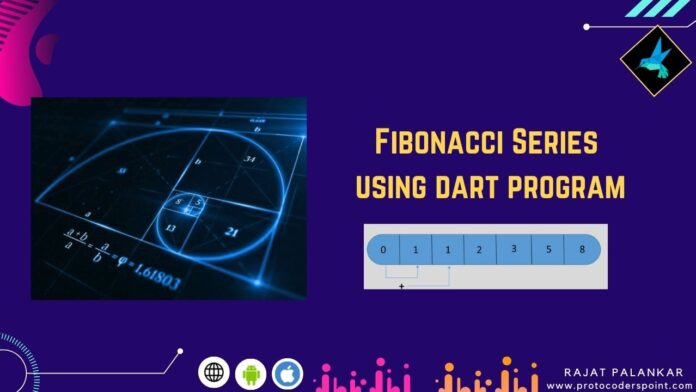Home Dart Dart program to generate fibonacci sequence number

# Dart program to generate fibonacci sequence number

0Hi, Welcome to Proto Coders Point, In this dart programming tutorial we will learn what is fibonacci number & write a dart program to generate fibonacci sequence number from 0 – N. It is one of the basic program every programming should know it.

## What is fibonacci sequence number?

In mathematic, the fibonacci series, is denoted by Fn.
Here, the next number will be the sum of previous two numbers.

Initial starting two numbers will be 0 & 1, denoted by F0 = 0, F1 = 1;

Simple mathematical formula to get Fn ‘ the next number’..

`Fn = F(n-1) +F(n-2)`

Therefore, the fib sequence looks like this:-

`0, 1, 1, 2, 3, 5, 8, 13, 21, 34, 55, 89, 144, ........ and so on up to Nth`

## How is fibonacci numbers generated?

Initially we have starting number which always start with 0 & 1 i.e F0 = 0, F1 = 1;

Now we perform sum of F0 + F1 = Fn.

i.e. F2 = F0 + F1.

Fibonacci Sequence flow animation example

Eg: initial value 0 & 1.

1st iteration: F2= F0 + F1
0 + 1
F2 = 1

2nd iteration: F3= F1 + F2
1 + 1
F3 = 2

3rd iteration: F4= F2 + F3
1 + 2
F4 = 3

4th iteration: F5= F3 + F4
2 + 3
F5 = 5

5th iteration: F6= F4 + F5
3 + 5
F6 = 8

and so on up to Nth

## How to implement fibonacci in dart? Fibonacci Algorithm.

`Step1: StartStep2: Declare initial variable f0 = 0, f1 = 1,f2, n, i =1;Step3: Read n from user, how many fibo sequence user wants to generate.Step4: Print f0 & f1Step5: Repeat until i < n         5.1: f2 = f0 + f1;         5.2: Print f2;         5.3: inter change value f0 = f1;                                     f1 = f2;         5.4: i ++;Step6: Stop.`

## Fibonacci program using dart

### Fibonacci program using loop

```void main() {
int n1=0,n2=1,n3;
print(n1);
print(n2);

for(int i = 2 ;i <= 16; i++){
n3 = n1+n2;
print('\$n3');
n1 = n2;
n2 = n3;
}
}```

### Fibonacci program using recursion

```int fibonacci(int n) => n <= 2 ? 1 : fibonacci(n - 2) + fibonacci (n - 1);

main() {
String output = "";
for (int i = 1; i <= 16; ++i) {
output += fibonacci(i).toString() + ", ";
}
print(output + "...");
}```

output

## What is a real world example of the Fibonacci numbers?

One of the best & easiliest way to understand fibonacci series used in real file application is, converting mile to kilometer and kilometer to mile.

Let’s now see the Fibonacci series :

0, 1, 1, 2, 3, 5, 8, 13, 21, 34, 55, 89, 144,…

For example :

1. Mile to kilometer conversion : If we take a number from Fibonacci series i.e., 8 then the kilometer value will be 12.874752 by formulae, which is nearly 13 by rounding.
2. Kilometer to mile conversion : If we take a number from Fibonacci series i.e., 89 as kilometer value then mile value will be 55.30203610912272 by formulae, which could be considered as 55 by rounding.

Finally in both conversions, the left element will be considered as mile value and right element will be considered as Kilometer value in the Fibonacci series.